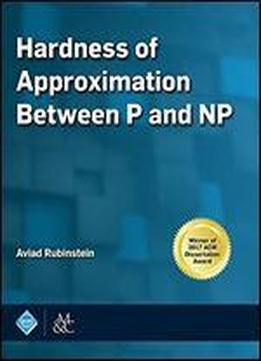# Hardness Of Approximation Between P And Np (acm Books) by Aviad Rubinstein / 2019 / English / EPUB

Nash equilibrium is the central solution concept in Game Theory. Since Nash's original paper in 1951, it has found countless applications in modeling strategic behavior of traders in markets, (human) drivers and (electronic) routers in congested networks, nations in nuclear disarmament negotiations, and more. A decade ago, the relevance of this solution concept was called into question by computer scientists, who proved (under appropriate complexity assumptions) that computing a Nash equilibrium is an intractable problem. And if centralized, specially designed algorithms cannot find Nash equilibria, why should we expect distributed, selfish agents to converge to one? The remaining hope was that at least approximate Nash equilibria can be efficiently computed. Understanding whether there is an efficient algorithm for approximate Nash equilibrium has been the central open problem in this field for the past decade. In this book, we provide strong evidence that even finding an approximate Nash equilibrium is intractable. We prove several intractability theorems for different settings (two-player games and many-player games) and models (computational complexity, query complexity, and communication complexity). In particular, our main result is that under a plausible and natural complexity assumption ("Exponential Time Hypothesis for PPAD"), there is no polynomial-time algorithm for finding an approximate Nash equilibrium in two-player games. The problem of approximate Nash equilibrium in a two-player game poses a unique technical challenge: it is a member of the class PPAD, which captures the complexity of several fundamental total problems, i.e., problems that always have a solution and it also admits a quasipolynomial time algorithm. Either property alone is believed to place this problem far below NP-hard problems in the complexity hierarchy having both simultaneously places it just above P, at what can be called the frontier of intractability. Indeed, the tools we develop in this book to advance on this frontier are useful for proving hardness of approximation of several other important problems whose complexity lies between P and NP: Brouwer's fixed point, market equilibrium, CourseMatch (A-CEEI), densest k-subgraph, community detection, VC dimension and Littlestone dimension, and signaling in zero-sum games.

views: 172